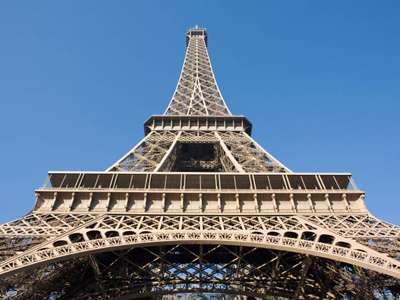How many centimetres tall is the Eiffel Tower? Find out in this quiz!

# Decimal Numbers 3 (Difficult)

Welcome to the third quiz in our Difficult series of Eleven Plus maths quizzes on Decimal Numbers. Now, you will know that metric measures (like tonnes, kilograms, metres and centimetres for example) are all based on decimal numbers. In this quiz we will test your ability to convert one to another by multiplying or dividing by 10, 100, 1,000, 10,000 or even 1,000,000.

• 1 tonne = 1,000 kg
• 1 kg = 1,000 grams
• 1 kilometre = 1,000 metres
• 1 m = 100 cm
• 1 cm = 10 millimetres

Armed with this information, you shouldn’t find the questions too difficult. Just take your time and read each on thoroughly before you choose your answer. Good luck!

1.
What was 0.00001 multiplied by if the answer is 10?
One thousand
Ten-thousand
One hundred-thousand
One million
For 0.00001 to become 10, the digits will have to move 6 places to the left. You will have to multiply 0.00001 by 1,000,000 - which has 6 zeros
2.
What was 10.7856 multiplied by if the answer is 107,856?
Ten-thousand
One-thousand
One hundred-thousand
One hundred
The rule for multiplying by a whole number beginning with 1 and followed by one or more zeros, e.g. multiplying by 10, 100, 1,000, ... is as follows: When multiplying by such a number, move the digits to the left by the same number of zeros as in the number: 10,000 × 10.7856 = 107,856: the digits have moved four places to the left = the number of zeros in ten-thousand
3.
A certain object weighs 9.374,808 tonnes. What is this in kilograms?
9,374.808 kg
93,748.08 kg
937.4808 kg
937,480.8 kg
There are 1,000 kg in 1 tonne, so in 9.374,808 tonnes there are 9.374,808 x 1,000 = 9,374.808 kg
4.
How many cubic centimetres are there in 2.8 cubic metres?
28,000 cm3
2,800,000 cm3
280,000 cm3
2,800 cm3
There are 100 cm in 1 m, so in 1 cubic m there are 100 × 100 x 100 = 1,000,000 cubic cm. In 2.8 cubic m there are 2.8 × 1,000,000 = 2,800,000 cm3
5.
What is 235 millimetres in metres?
0.0235 m
23.5 m
2.35 m
0.235 m
There are 1,000 mm in 1 m, so 235 mm ÷ 1,000 = 0.235 m
6.
What is 103.274 metres in millimetres?
10,327.4 mm
1,032.74 mm
103,274 mm
1,032,740 mm
There are 1,000 mm in 1 m, so in 103.274 m there are 1,000 × 103.274 = 103,274 mm
7.
How many grams are there in 26.872 kilograms?
26.872 g
2,687.2 g
268.72 g
26,872 g
There are 1,000 g in 1 kg, so in 26.872 kg there are 1,000 × 26.872 = 26,872 g
8.
How many grams are there in 2.397 tonnes?
239,700 g
2,397,000 g
23,970 g
2,397 g
There are 1,000 g in 1 kg, and 1,000 kg in 1 tonne. 2.397 x 1,000 x 1,000 = 2,397,000
9.
The Eiffel Tower is 0.324 km tall. How high is it in centimetres?
3,240,000 cm
324,000 cm
32,400 cm
3,240 cm
There are 1,000 m in 1 km, so in 0.324 km there are 0.324 × 1,000 = 324 m.
Next, there are 100 cm in 1 m, so in 324 m there are 324 x 100 = 32,400 cm
10.
What was 12,573 divided by if the answer is 0.12573?
One thousand
Ten-thousand
One hundred-thousand
One million
The rule for dividing by a whole number beginning with 1 and followed by one or more zeros, e.g. dividing by 10, 100, 1,000, ... is as follows: When dividing by such a number, move the digits to the right by the same number of zeros as in the number: 12,573 ÷ 100,000 = 0.12573: the digits have moved five places to the right = the number of zeros in one hundred-thousand
Author:  Frank Evans